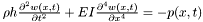# AMROC's beam solver - Documentation

A finite difference solver based on the equationwhere I is the moment of inertia divided by the beam width, p(x,t) the pressure loading, and w(x,t) the displacement. Typical boundary conditions at both ends, such as fixed, freely moving, momentum-free, are supported. The equation is transformed into a first-order system and temporally discretized with Crank-Nicholson. A straightforward LR decomposition is used for direct solution of the set of linear equations.

Generated on Fri Aug 24 13:01:04 2007 for AMROC's Beam solver - by1.4.7